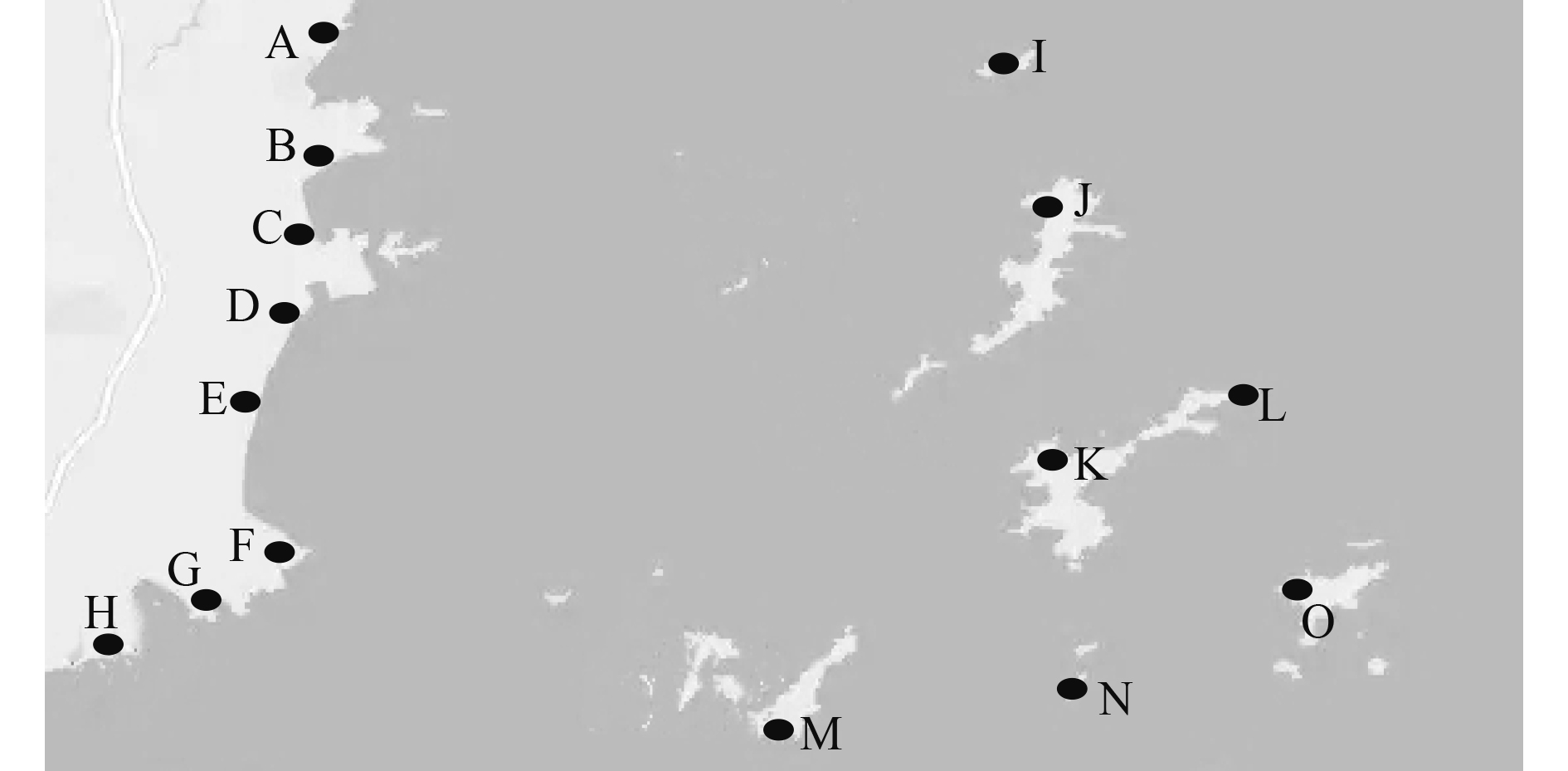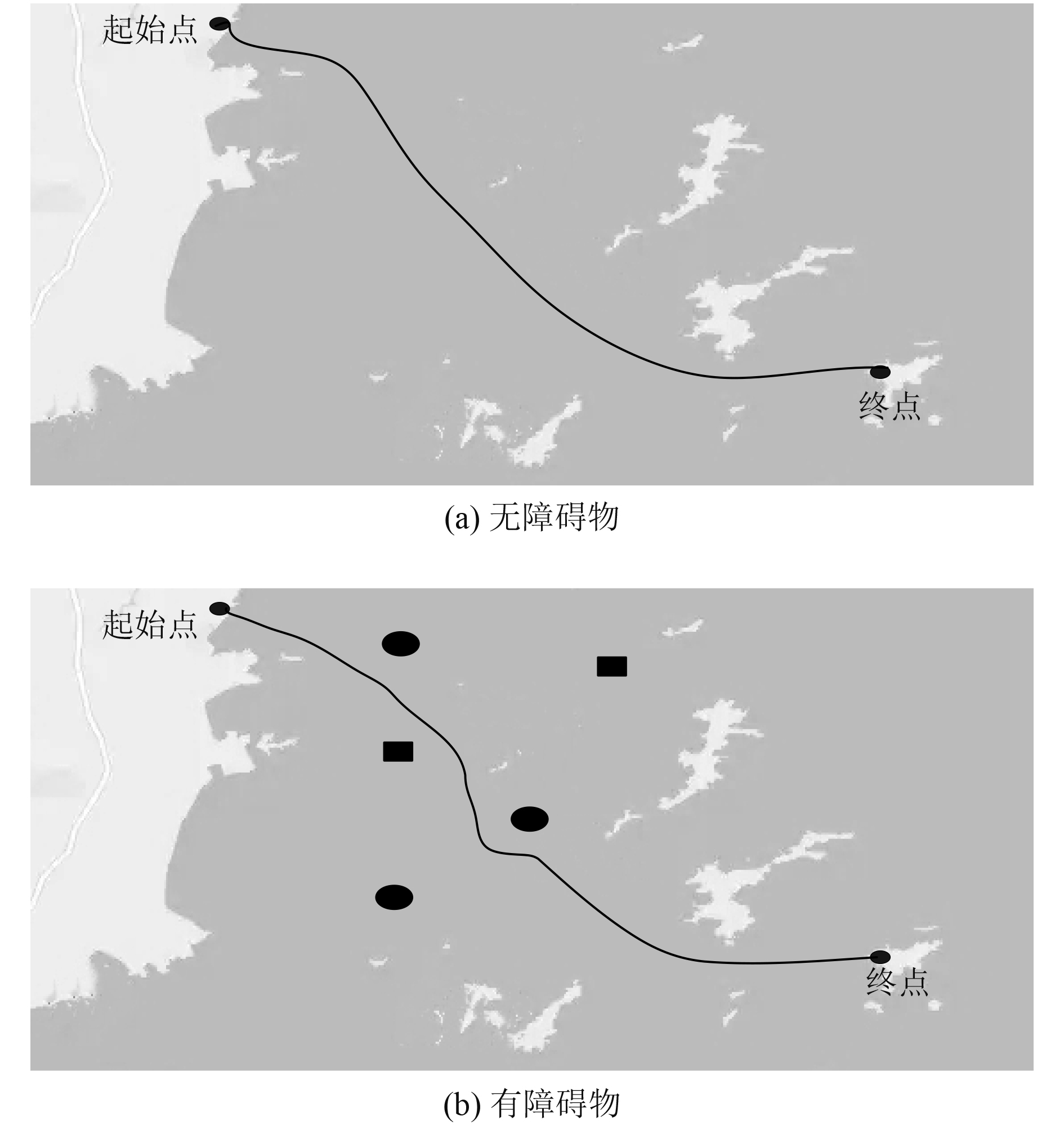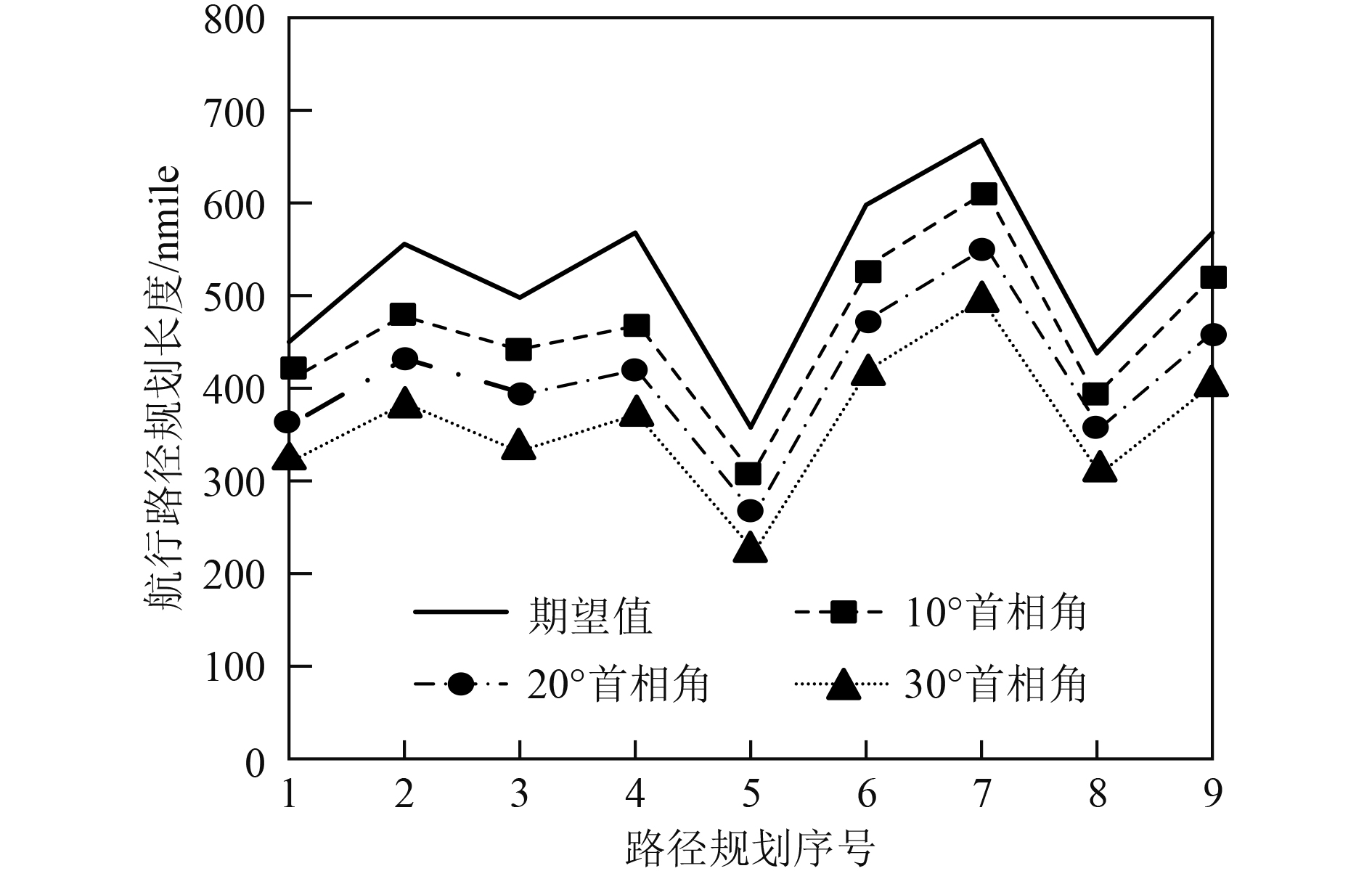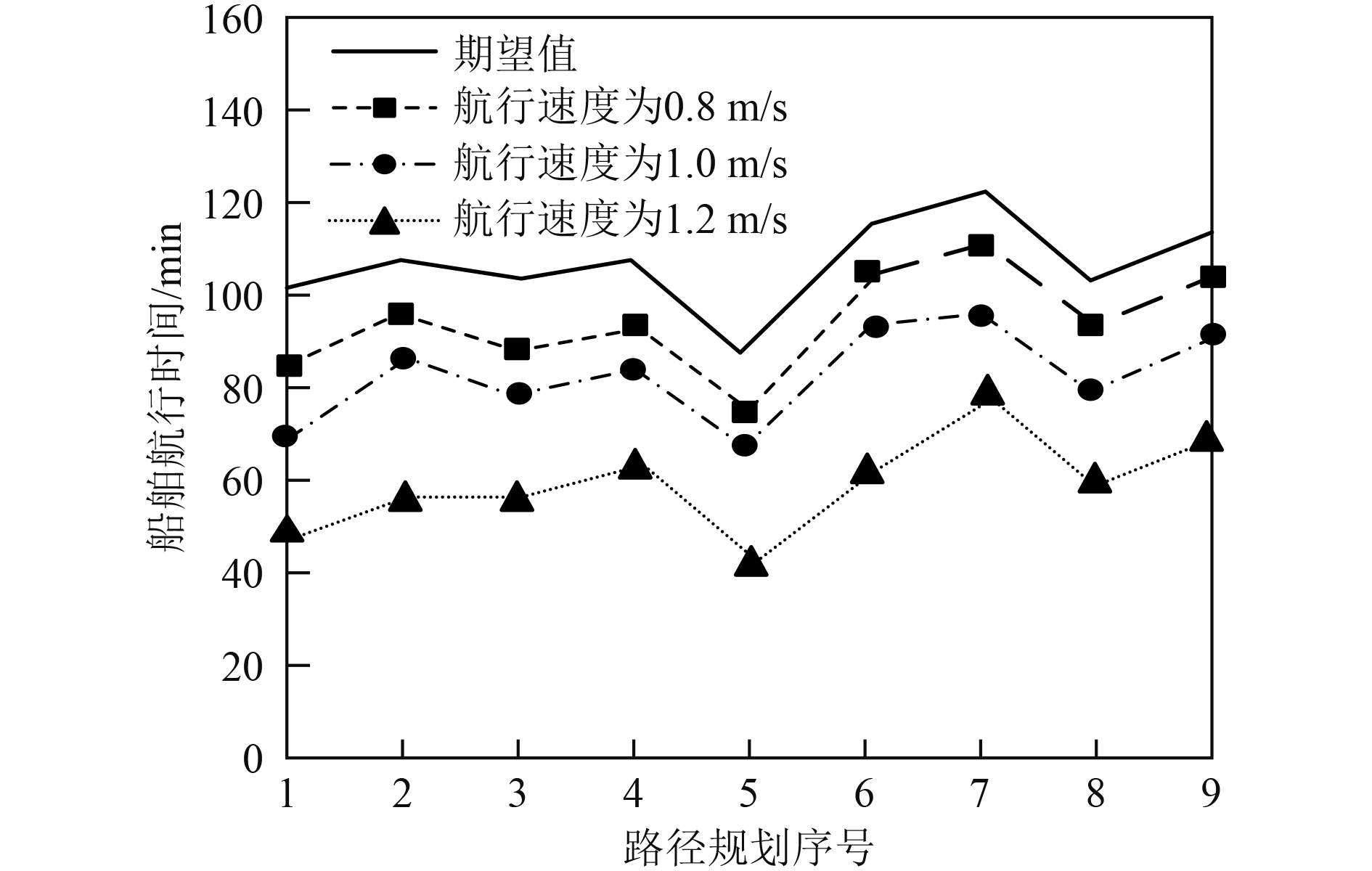﻿ 基于改进神经网络的船舶航行路径规划
 舰船科学技术2022, Vol. 44Issue (14): 57-60    DOI: 10.3404/j.issn.1672-7649.2022.14.013PDF

Research on ship navigation path planning based on improved neural network
XU Zhi-yuan
School of Navigation and Naval Architecture, Dalian Ocean University, Dalian 116023, China
Abstract: In order to improve the economy and safety of ship navigation path, a ship navigation path planning method based on improved neural network is proposed. The improved neural network method is used to solve the established ship navigation path planning model, the modified linear unit relu function is selected to replace the original activation function, the gradient descent method is selected to update the neural network weight, the root mean square back propagation algorithm is used to optimize the neural network, and the ship navigation path planning results are output. Collision detection is carried out by using the results of ship navigation path planning to avoid collision of ship navigation path. The experimental results show that this method can effectively plan the ship's navigation path, and the planned length of the ship's navigation path meets the expected requirements, without collision, and meets the economic and safety requirements of the ship's navigation.
Key words: improved neural network     ship     sailing     path planning     gradient descent     activation function
0 引　言

1 船舶航行路径规划 1.1 构建船舶航行路径规划模型

$S$ 表示船舶，设船舶属于二维空间中存在航行运动的刚体，通过小波滤波处理后，获取的船舶质心坐标表示为(xe,ye)，船舶航行区域表示为 ${\Omega _f}$ ，由船舶航行区域组成的水域表示为 ${\Omega _0}$ 。设水域中存在危险区域数量为 $n$ ，为了满足船舶航行路径规划过程中不存在障碍物的条件，船舶航行路径规划时，路径 $L$ 中的全部节点与障碍物不存在交集。将船舶航行路径规划问题描述为：寻找一个二元函数f(x,y)=0于二维坐标系中，依据船舶航行路径与障碍物间的距离要求，建立船舶航行路径规划模型表达式如下

 $y=d\left( {{X_S}} \right) = \mathop {\min }\limits_{{X_0} \in {\Omega _0}} \left\| {{X_S} - {X_0}} \right\|。$ (1)

1.2 改进神经网络的船舶航行规划模型求解

BP神经网络是由输入层、隐含层、输出层构成的多层前馈网络。输出层与隐含层的权重增量求解公式如下：

 $\Delta {w_{jk}} = \left( {{d_0}\left( n \right) - {y_0}\left( n \right)} \right){\delta _j}\eta {y_j}f{\left( {ne{t_k}} \right)^\prime } 。$ (2)

 $\Delta {w_{ij}} = \left( {\sum\limits_{k = 1}^L {{\delta _k}\eta {w_{jk}}} } \right){x_i}\eta f{\left( {ne{t_j}} \right)^\prime } 。$ (3)

 $E = \frac{1}{2}\sum\limits_{o = 1}^q {\eta {{\left( {{d_o}\left( n \right) - {y_o}\left( n \right)} \right)}^2}} 。$ (4)

 $f\left( x \right) = \left\{ {\begin{array}{*{20}{c}} {x,x > 0} ，\\ {\lambda x,x \leqslant 0} 。\end{array}} \right.$ (5)

 ${w_{i + 1}} = {w_i} - \alpha \eta \frac{{\partial C}}{{\partial {w_i}}} 。$ (6)

 $M\left( {w,{t_0}} \right) = 0.8M\left( {w,{t_0} - 1} \right) + 0.2{\left( {\eta \frac{{\partial E}}{{\partial {w^{{t_0}}}}}} \right)^2}。$ (7)

1.3 船舶航行路径碰撞检测

 $C_R^t = f\left( {T_R^t} \right) 。$ (8)

 $T_R^t = \sum\limits_{m = 1}^{{G_R}} {O_m^t + {\theta _{TR}}}。$ (9)

 $O_m^t = f\left( {I_m^t} \right)。$ (10)

 $I_m^t = {w_{xm}}x + {w_{ym}}y\left( {v_{Rx}^t + v_{Ry}^t} \right) + \theta _m^t 。$ (11)

 $E = \sum\limits_{k = 1}^Q {\sum\limits_{j = 1}^Q {{C_{{R_{kj}}}}} }。$ (12)

2 实验结果与分析图 1 船舶路径规划海域 Fig. 1 Ship path planning sea area图 2 船舶航行路径规划结果 Fig. 2 The result of ship navigation path planning图 3 船舶航行路径规划长度 Fig. 3 The planned length of the ship's navigation path图 4 船舶航行时间 Fig. 4 Ship sailing time
3 结　语

  陈秋莲, 郑以君, 蒋环宇, 等. 基于神经网络改进粒子群算法的动态路径规划[J]. 华中科技大学学报(自然科学版), 2021, 49(2): 51-55.  祁林, 渠俊锋, 司文杰, 等. 基于RBF神经网络的水面船舶轨迹跟踪控制[J]. 船舶工程, 2021, 43(1): 95-101+118.  随博文, 黄志坚, 姜宝祥, 等. 基于深度Q网络的水面无人艇路径规划算法[J]. 上海海事大学学报, 2020, 41(3): 1-5+116.  胡玉可, 夏维, 胡笑旋, 等. 基于循环神经网络的船舶航迹预测[J]. 系统工程与电子技术, 2020, 42(4): 871-877. DOI:10.3969/j.issn.1001-506X.2020.04.18  朱大奇, 朱婷婷, 颜明重. 基于改进神经网络的多AUV全覆盖路径规划[J]. 系统仿真学报, 2020, 32(8): 1505-1514.  刘长德, 顾宇翔, 张进丰. 基于小波滤波和LSTM神经网络的船舶运动极短期预报研究[J]. 船舶力学, 2021, 25(3): 299-310. DOI:10.3969/j.issn.1007-7294.2021.03.005  向金林, 王鸿东, 欧阳子路, 等. 基于改进双向RRT的无人艇局部路径规划算法研究[J]. 中国造船, 2020, 61(1): 157-166. DOI:10.3969/j.issn.1000-4882.2020.01.016  童帮裕, 胡坚堃. 基于改进蚁群算法的船舶冰区航行路径规划[J]. 中国航海, 2020, 43(1): 24-28. DOI:10.3969/j.issn.1000-4653.2020.01.005  郝启润, 吴韵哲, 吴浩峻, 等. 基于改进A~*GBNN算法的AUV路径规划[J]. 船舶工程, 2020, 42(9): 128-132+156.